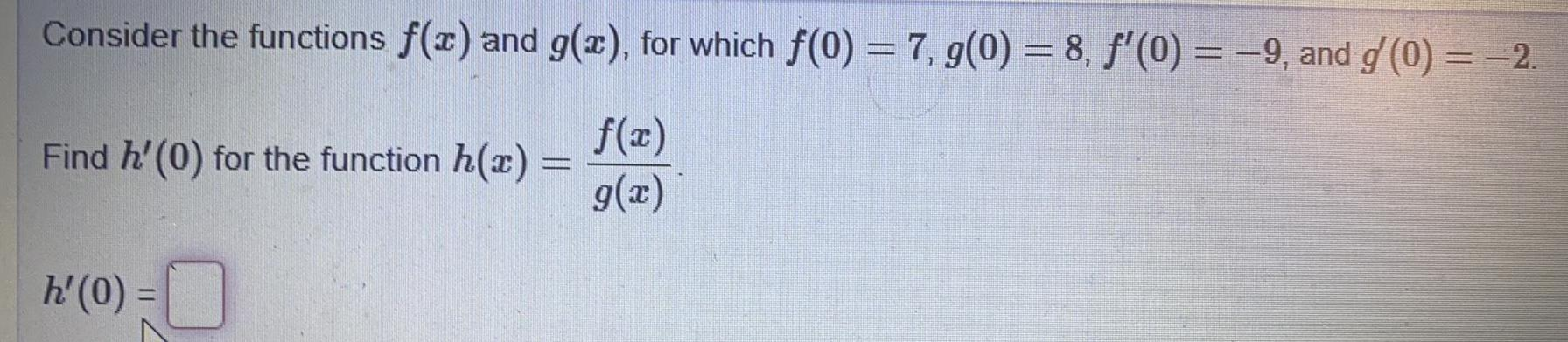Question:

# Consider the functions f(x) and g(x), for which f(0) = 7,

Last updated: 7/30/2022Consider the functions f(x) and g(x), for which f(0) = 7, g(0) = 8, f'(0) = -9, and g'(0) = -2. f(x) g(x) Find h'(0) for the function h(x) = h'(0) =